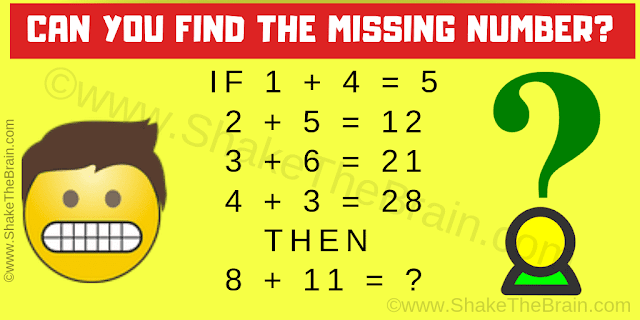## Saturday, November 23, 2019

This is a very interesting maths logic equations puzzle which will challenge your brain. Solving this maths logic puzzle will help you to improve your logical reasoning skills. You can also test your maths skills. In this maths logic question, you are shown some logic number equations. Can you find the logical relationship in these number equations and then find the value of the missing number?Can you find the value of the missing number?
The answer to this "Interesting Maths Equation Puzzle", can be viewed by clicking on the answer button.

#### 1 comment:

1.I wuz so confussed